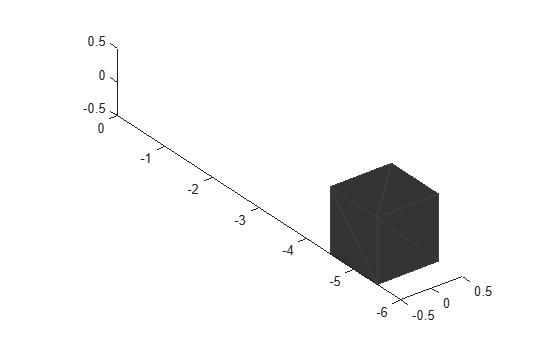# show

Display the mesh as a patch on the current axes

## Syntax

``show(mesh)``
``show(mesh,ax)``
``ax = show(mesh)``

## Description

example

````show(mesh)` displays the `extendedObjectMesh` as a patch on the current axes. If there are no active axes, the function creates new axes.```
````show(mesh,ax)` displays the object mesh as a patch on the axes `ax`.```
````ax = show(mesh)` optionally outputs the handle to the axes where the mesh was plotted.```

## Examples

collapse all

Create an `extendedObjectMesh` object and translate the object.

Construct a cuboid mesh.

`mesh = extendedObjectMesh('cuboid');`

Translate the mesh by 5 units along the negative y axis.

`mesh = translate(mesh,[0 -5 0]);`

Visualize the mesh.

```ax = show(mesh); ax.YLim = [-6 0];```## Input Arguments

collapse all

Extended object mesh, specified as an `extendedObjectMesh` object.

Current axes, specified as an `axes` object.

Introduced in R2020b

## SupportGet trial now